Select Page

# Conversion from Mealy to Moore Machine

Conversion from Mealy to Moore Machine is little bit complex than conversion of Moore to Mealy Machine. There are two cases while conversion of Mealy to Moore Machine.

Case 01: When the every entering Input in Mealy state providing the same output. Then simply place that output with state.

Case 02: When the every entering Input in any particular Mealy state is providing the different output then we cannot represent more than one output in one state of Moore Machine. In this case that particular state is duplicated.

## Examples of Mealy to Moore Machine Conversion

Let explain some examples of Mealy to Moore Machine Conversions.

### Example 01

Consider the following Mealy Machine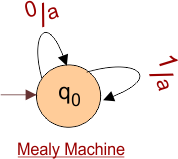As in the above Mealy Machine,

• q0 is the start state, (0,1) are inputs and “a” is the output.
• Every entering input in the state q0 having the similar output “a”.
• So, simply cut the output “a” over arrow and place along with state “q0”.

After conversion the Moore Machine is given under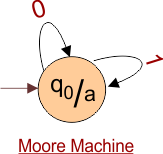### Example 02

Consider the following Mealy Machine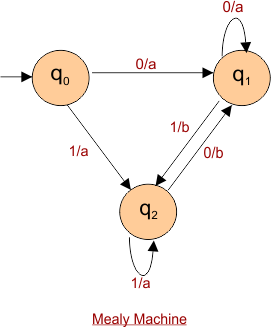At point q0,

There is no arrow is entering into the state q0. So, the output for the q0 is null. As given Below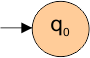At point q1,

There are three arrows are entering into the state q1. Arrow from q0 and q1 has the input “0” with output “a” and Arrow from q2 has the input “1” with “b” output.

Output of 2 types (0,1) are entering into state q1, So One duplicate of q1 is generated with different output.

So, the output of type “0” is placed along with state q1. And Output of type “1” is placed along with duplicated state (q1) as given below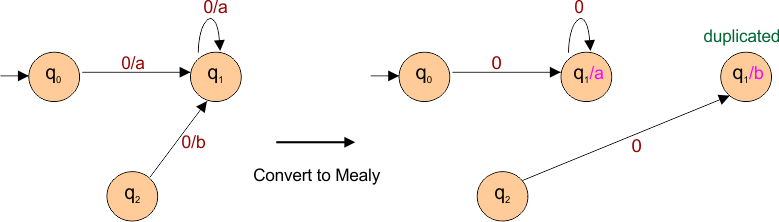Further Proceeding of above,  Now make the transition from both states (original q1 and duplicate q1) for input 0 and 1.

• For input “0” transition goes to q1 with “a”.
• For input “1” transition goes to q2 with output “b”.

As given below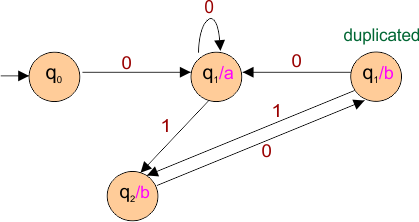Now remaining state is only q2 which has to discuss.

There are three arrows are entering into the state q2. Arrow from q0 and q2 (itself) has the input “0” with output “a” and Arrow from q2 has the input “1” with “b” output.

Output of 2 types (0,1) are entering into state q2, So One duplicate state of q2 is generated.

By completing the transition from both states (original q2 and duplicate q2) for input 0 and 1, the final Mealy machine is given below

As shown in following diagram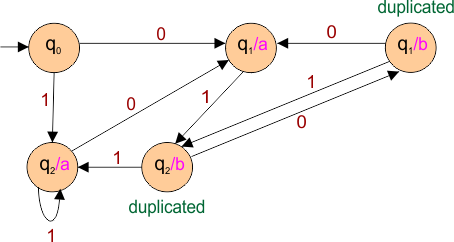Important.

If “M” is the number of states and “N” is number of outputs in Mealy Machine, then maximum number of States in Mealy Machine will be “M” multiply by “N”.

Suppose if 3 number of states and 2 number of outputs in Mealy, then 6 will be the maximum state in Moore Machine

Help Other’s By Sharing…